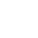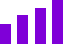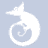quizzes  Forums

# Math Calculations

45 played - 9 yrs ago
4t+5=37 t=8 4(8)=32+5=377 QUESTIONS
26%1
Which of these questions is true?

2
Which of these questions is false?

3
If 4n+4=20 then n=

4
If 5v-3=22 then v=

5
If 6p+6=42 then p=

6
If 5m-8=57 then m=

7
If 2d+1=9 then d=